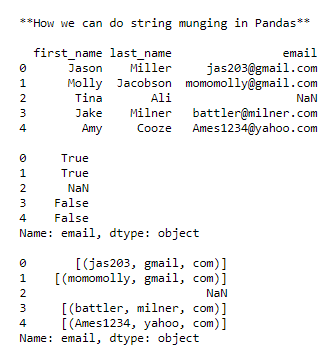Skip to content
Related Articles
String Munging In Pandas Dataframe
• Last Updated : 03 Jan, 2021

In this article, we are going to learn about String Munging In Pandas Dataframe. Munging is known as cleaning up anything which was messy by transforming them. In technical terms, we can say that transforming the data in the database into a useful form.

Example: “no-one@example.com”, becomes “no-one at example dot com”

Approach:

Step 1: import the library

## Python3

 `import` `pandas as pd``import` `numpy as np``import` `re as re`

Step 2: creating Dataframe

Now create a dictionary and pass it through pd.DataFrame to create a Dataframe.

## Python3

 `raw_data ``=` `{``"first_name"``: [``"Jason"``, ``"Molly"``, ``"Tina"``, ``"Jake"``, ``"Amy"``],``            ``"last_name"``: [``"Miller"``, ``"Jacobson"``, ``"Ali"``, ``"Milner"``, ``"Cooze"``],``            ``"email"``: [``"jas203@gmail.com"``, ``"momomolly@gmail.com"``, np.NAN,``                      ``"battler@milner.com"``, ``"Ames1234@yahoo.com"``]}`` ` `df ``=` `pd.DataFrame(raw_data, columns``=``[``"first_name"``, ``"last_name"``, ``"email"``])``print``()``print``(df)`

Step 3: Applying Different Munging Operation

First, check that in feature “email” which string contains “Gmail”.

## Python3

 `print``(df[``"email"``].``str``.contains(``"gmail"``))`

Now we want to separate the email into parts such that characters before “@” becomes one string and after and before “.” becomes one. At last, the remaining becomes the one string.

## Python3

 `pattern ``=` `"([A-Z0-9._%+-]+)@([A-Z0-9.-]+)\.([A-Z]{2,4})"``print``(df[``"email"``].``str``.findall(pattern, flags``=``re.IGNORECASE))`

Below is the implementation:

## Python3

 `def` `ProjectPro_Ex_136():`` ` `    ``print``()``    ``print``(``'**How we can do string munging in Pandas**'``)`` ` `    ``# loading libraries``    ``import` `pandas as pd``    ``import` `numpy as np``    ``import` `re as re`` ` `    ``# Creating dataframe``    ``raw_data ``=` `{``'first_name'``: [``'Jason'``, ``'Molly'``, ``'Tina'``, ``'Jake'``, ``'Amy'``],``                ``'last_name'``: [``'Miller'``, ``'Jacobson'``, ``'Ali'``, ``'Milner'``, ``'Cooze'``],``                ``'email'``: [``'jas203@gmail.com'``, ``'momomolly@gmail.com'``, np.NAN,``                          ``'battler@milner.com'``, ``'Ames1234@yahoo.com'``]}`` ` `    ``df ``=` `pd.DataFrame(raw_data, columns``=``[``'first_name'``, ``'last_name'``, ``'email'``])``    ``print``()``    ``print``(df)`` ` `    ``# Let us find Which string within the ``    ``# email column contains ‘gmail’``    ``print``()``    ``print``(df[``'email'``].``str``.contains(``'gmail'``))`` ` `    ``# Create a daily expression pattern that``    ``# breaks apart emails``    ``pattern ``=` `'([A-Z0-9._%+-]+)@([A-Z0-9.-]+)\\.([A-Z]{2,4})'`` ` `    ``# Find everything in df.email that contains``    ``# that pattern``    ``print``()``    ``print``(df[``'email'``].``str``.findall(pattern, flags``=``re.IGNORECASE))`` ` ` ` `ProjectPro_Ex_136()`

Output:Attention geek! Strengthen your foundations with the Python Programming Foundation Course and learn the basics.

To begin with, your interview preparations Enhance your Data Structures concepts with the Python DS Course. And to begin with your Machine Learning Journey, join the Machine Learning – Basic Level Course

My Personal Notes arrow_drop_up